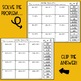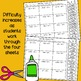# Powers of 10 ActivitySubject
Resource Type
File Type

PDF

(14 MB|9 pages)
Standards
• Product Description
• StandardsNEW

Students will practice multiplying and dividing by powers of 10 with this set of 4 matching activities. Includes whole numbers and decimals. Practicing the powers of ten is fun with this alternative to traditional worksheets!

This resource includes:

• 4 different matching cut and paste activity sheets
• Each sheet has 12 different problems for students to solve (and 12 matching answers)
• answer keys for each page
• teacher directions and information

The difficulty level increases as students work through the four different activities:

• sheet #1- Multiply whole numbers by powers of 10
• sheet #2- Multiply decimals by powers of 10
• sheet #3- Divide whole numbers by powers of 10
• sheet #4- Divide decimals by powers of 10

This resource provides a great way for students to get valuable practice with multiplying and dividing by powers of ten and allows them to self correct when they make mistakes. If they solve a problem, and the solution isn't in the answer list, they can go back to the problem and correct their errors.

This resource works great as:

• a whole class activity or practice
• part of small group math instruction or interventions
• math center or station activities
• homework

This is a great resource to add to your collection of powers of 10 and place value activities!

You may also like my:

Metric Measurement Conversions Activity

Subtracting Decimals Math Clips

Multiplying Decimals Math Clips

Order of Operations Math Clips

Long Division Math Clips

Follow me and be notified when new products are added to my store. New products are always 50% off for the first 24 hours they are posted!

Explain patterns in the number of zeros of the product when multiplying a number by powers of 10, and explain patterns in the placement of the decimal point when a decimal is multiplied or divided by a power of 10. Use whole-number exponents to denote powers of 10.
Total Pages
9 pages
Included
Teaching Duration
N/A
Report this Resource to TpT
Reported resources will be reviewed by our team. Report this resource to let us know if this resource violates TpT’s content guidelines.